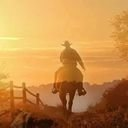### Reasons for signing

See why other supporters are signing, why this petition is important to them, and share your reason for signing (this will mean a lot to the starter of the petition).JustusVEGAN Bruns
2 years ago
<3<<3<3<3VEGANVEGANVEGANVEANVEGANVEGANVEGANVEGANVEGANVEGANVEGANVEGANVEGANVEGANVEGANVEGANVEGANVEGANVEGANVEGANVEGANVEGANVEANVEGANVEGANVEGANVEGANVEGANVEGANVEGANVEGANVEGANVEGANVEGANVEGANVEGANVEGANVEGANVEGANVEGANVEGAN<33<3<3<3<3<3<333<<33<3<<3<3<3<3<3<3<3<3<333<<3<3<3<3<3<3<3<3<3<33<3<<3<3<3<3<3<3<33<3<3<33<3<3<3<3<<3<3<33<<3<3<3<3<33<<3<3<3<3<3<3<3<3<3<3<3<3<3<3<3<<3<<3<3<3<3<3<3<3<3<<3<3<3<3<3<3<3<3<3<3<3<3<3<33VEGANVEGANVEGANVEGANVEGANVEGANVEGANVEANVEGANVEGANVEGANVEGANVEGANVEGANVEGANVEGANVEGANVEGANVEGANVEGANVEGANVEGANVEGANVEGANVEGANVEGANVEANVEGANVEGANVEGANVEGANVEGANVEGANVEGANVEGANVEGANVEGANVEGANVEGANVEGANVEGANVEGANVEGANVEGANVEGAN<33<3<3<3<3<3<333<<33<3<<3<3<3<3<3<3<3<3<333<<3<3<3<3<3<3<3<3<3<33<3<<3<3<3<3<3<3<33<3<3<33<3<3<3<3<<3<3<33<<3<3<3<3<33<<3<3<3<3<3<3<3<3<3<3<3<3<3<3<3<<3<<3<3<3<3<3<3<3<3<<3<3<3<3<3<3<3<3<3<3<3<3<3<33VEGANVEGANVEGANVEGANVEGANVEGANVEGANVEANVEGANVEGANVEGANVEGANVEGANVEGANVEGANVEGANVEGANVEGANVEGANVEGANVEGANVEGANVEGANVEGANVEGANVEGANVEANVEGANVEGANVEGANVEGANVEGANVEGANVEGANVEGANVEGANVEGANVEGANVEGANVEGANVEGANVEGANVEGANVEGANVEGAN<33<3<3<3<3<3<333<<33<3<<3<3<3<3<3<3<3<3<333<<3<3<3<3<3<3<3<3<3<33<3<<3<3<3<3<3<3<33<3<3<33<3<3<3<3<<3<3<33<<3<3<3<3<33<<3<3<3<3<3<3<3<3<3<3<3<3<3<3<3<<3<<3<3<3<3<3<3<3<3<<3<3<3<3<3<3<3<3<3<3<3<3<3<33VEGANVEGANVEGANVEGANVEGANVEGANVEGANVEANVEGANVEGANVEGANVEGANVEGANVEGANVEGANVEGANVEGANVEGANVEGANVEGANVEGANVEGANVEGANVEGANVEGANVEGANVEANVEGANVEGANVEGANVEGANVEGANVEGANVEGANVEGANVEGANVEGANVEGANVEGANVEGANVEGANVEGANVEGANVEGANVEGAN<33<3<3<3<3<3<333<<33<3<<3<3<3<3<3<3<3<3<333<<3<3<3<3<3<3<3<3<3<33<3<<3<3<3<3<3<3<33<3<3<33<3<3<3<3<<3<3<33<<3<3<3<3<33<<3<3<3<3<3<3<3<3<3<3<3<3<3<3<3<<3<<3<3<3<3<3<3<3<3<<3<3<3<3<3<3<3<3<3<3<3<3<3<33VEGANVEGANVEGANVEGANVEGANVEGANVEGANVEANVEGANVEGANVEGANVEGANVEGANVEGANVEGANVEGANVEGANVEGANVEGANVEGANVEGANVEGANVEGANVEGANVEGANVEGANVEANVEGANVEGANVEGANVEGANVEGANVEGANVEGANVEGANVEGANVEGANVEGANVEGANVEGANVEGANVEGANVEGANVEGANVEGAN<33<3<3<3<3<3<333<<33<3<<3<3<3<3<3<3<3<3<333<<3<3<3<3<3<3<3<3<3<33<3<<3<3<3<3<3<3<33<3<3<33<3<3<3<3<3<3<3<3<3<3<3<3<3<3<3<3<3<3<3<3<3<<<33<33333
<3<3<33<<3<3<3<3<33333<3<3<3<3<3<333333VEGABEGANVEGANVEGANVEGAN<3<3<3<3<3<333<3<3<333<3<3VEGANVEGANVEGANVEGANVEGAN<3<3<3<3<3<3<3VEGANVEGANVEGANVEGAN<3<3<3<33VEGANVEGANVEGANVEGANVEGAN<3<33<<3<3<3<3333333<3<3<3<3<3<3<<3<3<3<3<33<3VEGAVEGANVEGANVEGANVEGANJustusVEGAN Bruns
2 years ago
VEGANVEGANVEGANVEANVEGANVEGANVEGANVEGANVEGANVEGANVEGANVEGANVEGANVEGANVEGANVEGANVEGANVEGANVEGANVEGANVEGANVEGANVEANVEGANVEGANVEGANVEGANVEGANVEGANVEGANVEGANVEGANVEGANVEGANVEGANVEGANVEGANVEGANVEGANVEGANVEGAN<33<3<3<3<3<3<333<<33<3<<3<3<3<3<3<3<3<3<333<<3<3<3<3<3<3<3<3<3<33<3<<3<3<3<3<3<3<33<3<3<33<3<3<3<3<<3<3<33<<3<3<3<3<33<<3<3<3<3<3<3<3<3<3<3<3<3<3<3<3<<3<<3<3<3<3<3<3<3<3<<3<3<3<3<3<3<3<3<3<3<3<3<3<33VEGANVEGANVEGANVEGANVEGANVEGANVEGANVEANVEGANVEGANVEGANVEGANVEGANVEGANVEGANVEGANVEGANVEGANVEGANVEGANVEGANVEGANVEGANVEGANVEGANVEGANVEANVEGANVEGANVEGANVEGANVEGANVEGANVEGANVEGANVEGANVEGANVEGANVEGANVEGANVEGANVEGANVEGANVEGANVEGAN<33<3<3<3<3<3<333<<33<3<<3<3<3<3<3<3<3<3<333<<3<3<3<3<3<3<3<3<3<33<3<<3<3<3<3<3<3<33<3<3<33<3<3<3<3<<3<3<33<<3<3<3<3<33<<3<3<3<3<3<3<3<3<3<3<3<3<3<3<3<<3<<3<3<3<3<3<3<3<3<<3<3<3<3<3<3<3<3<3<3<3<3<3<33VEGANVEGANVEGANVEGANVEGANVEGANVEGANVEANVEGANVEGANVEGANVEGANVEGANVEGANVEGANVEGANVEGANVEGANVEGANVEGANVEGANVEGANVEGANVEGANVEGANVEGANVEANVEGANVEGANVEGANVEGANVEGANVEGANVEGANVEGANVEGANVEGANVEGANVEGANVEGANVEGANVEGANVEGANVEGANVEGAN<33<3<3<3<3<3<333<<33<3<<3<3<3<3<3<3<3<3<333<<3<3<3<3<3<3<3<3<3<33<3<<3<3<3<3<3<3<33<3<3<33<3<3<3<3<<3<3<33<<3<3<3<3<33<<3<3<3<3<3<3<3<3<3<3<3<3<3<3<3<<3<<3<3<3<3<3<3<3<3<<3<3<3<3<3<3<3<3<3<3<3<3<3<33VEGANVEGANVEGANVEGANVEGANVEGANVEGANVEANVEGANVEGANVEGANVEGANVEGANVEGANVEGANVEGANVEGANVEGANVEGANVEGANVEGANVEGANVEGANVEGANVEGANVEGANVEANVEGANVEGANVEGANVEGANVEGANVEGANVEGANVEGANVEGANVEGANVEGANVEGANVEGANVEGANVEGANVEGANVEGANVEGAN<33<3<3<3<3<3<333<<33<3<<3<3<3<3<3<3<3<3<333<<3<3<3<3<3<3<3<3<3<33<3<<3<3<3<3<3<3<33<3<3<33<3<3<3<3<<3<3<33<<3<3<3<3<33<<3<3<3<3<3<3<3<3<3<3<3<3<3<3<3<<3<<3<3<3<3<3<3<3<3<<3<3<3<3<3<3<3<3<3<3<3<3<3<33VEGANVEGANVEGANVEGANVEGANVEGANVEGANVEANVEGANVEGANVEGANVEGANVEGANVEGANVEGANVEGANVEGANVEGANVEGANVEGANVEGANVEGANVEGANVEGANVEGANVEGANVEANVEGANVEGANVEGANVEGANVEGANVEGANVEGANVEGANVEGANVEGANVEGANVEGANVEGANVEGANVEGANVEGANVEGANVEGAN<33<3<3<3<3<3<333<<33<3<<3<3<3<3<3<3<3<3<333<<3<3<3<3<3<3<3<3<3<33<3<<3<3<3<3<3<3<33<3<3<33<3<3<3<3<3<3<3<3<3<3<3<3<3<3<3<3<3<3<3<3<3<3<3<3<33<3<3<333<3<3<3<3333<<3<3<3<3<3<3<3<3<3<3<<3<3<3<33<3<<3<<3<33333333<3<3<33<3<3<3<3<<33<<33<<3<3<3<3<3<3<3<3<3<3<3<3<3<33333<3<33<3<3<3<33<3<<3<3<3<3<3<33<3<<33<3<3<3<<3<33<<33<<333333333<3<333<333<333<3<3<3<3<33<33<33<<3<33<3<3<3<3<3<3<33<33333333<3JustusVEGAN Bruns
2 years ago
VEGANVEGANVEGANVEGANVEGANVEGANVEGANVEANVEGANVEGANVEGANVEGANVEGANVEGANVEGANVEGANVEGANVEGANVEGANVEGANVEGANVEGANVEGANVEGANVEGANVEGANVEANVEGANVEGANVEGANVEGANVEGANVEGANVEGANVEGANVEGANVEGANVEGANVEGANVEGANVEGANVEGANVEGANVEGANVEGAN<33<3<3<3<3<3<333<<33<3<<3<3<3<3<3<3<3<3<333<<3<3<3<3<3<3<3<3<3<33<3<<3<3<3<3<3<3<33<3<3<33<3<3<3<3<<3<3<33<<3<3<3<3<33<<3<3<3<3<3<3<3<3<3<3<3<3<3<3<3<<3<<3<3<3<3<3<3<3<3<<3<3<3<3<3<3<3<3<3<3<3<3<3<33VEGANVEGANVEGANVEGANVEGANVEGANVEGANVEANVEGANVEGANVEGANVEGANVEGANVEGANVEGANVEGANVEGANVEGANVEGANVEGANVEGANVEGANVEGANVEGANVEGANVEGANVEANVEGANVEGANVEGANVEGANVEGANVEGANVEGANVEGANVEGANVEGANVEGANVEGANVEGANVEGANVEGANVEGANVEGANVEGAN<33<3<3<3<3<3<333<<33<3<<3<3<3<3<3<3<3<3<333<<3<3<3<3<3<3<3<3<3<33<3<<3<3<3<3<3<3<33<3<3<33<3<3<3<3<<3<3<33<<3<3<3<3<33<<3<3<3<3<3<3<3<3<3<3<3<3<3<3<3<<3<<3<3<3<3<3<3<3<3<<3<3<3<3<3<3<3<3<3<3<3<3<3<33VEGANVEGANVEGANVEGANVEGANVEGANVEGANVEANVEGANVEGANVEGANVEGANVEGANVEGANVEGANVEGANVEGANVEGANVEGANVEGANVEGANVEGANVEGANVEGANVEGANVEGANVEANVEGANVEGANVEGANVEGANVEGANVEGANVEGANVEGANVEGANVEGANVEGANVEGANVEGANVEGANVEGANVEGANVEGANVEGAN<33<3<3<3<3<3<333<<33<3<<3<3<3<3<3<3<3<3<333<<3<3<3<3<3<3<3<3<3<33<3<<3<3<3<3<3<3<33<3<3<33<3<3<3<3<<3<3<33<<3<3<3<3<33<<3<3<3<3<3<3<3<3<3<3<3<3<3<3<3<<3<<3<3<3<3<3<3<3<3<<3<3<3<3<3<3<3<3<3<3<3<3<3<33VEGANVEGANVEGANVEGANVEGANVEGANVEGANVEANVEGANVEGANVEGANVEGANVEGANVEGANVEGANVEGANVEGANVEGANVEGANVEGANVEGANVEGANVEGANVEGANVEGANVEGANVEANVEGANVEGANVEGANVEGANVEGANVEGANVEGANVEGANVEGANVEGANVEGANVEGANVEGANVEGANVEGANVEGANVEGANVEGAN<33<3<3<3<3<3<333<<33<3<<3<3<3<3<3<3<3<3<333<<3<3<3<3<3<3<3<3<3<33<3<<3<3<3<3<3<3<33<3<3<33<3<3<3<3<<3<3<33<<3<3<3<3<33<<3<3<3<3<3<3<3<3<3<3<3<3<3<3<3<<3<<3<3<3<3<3<3<3<3<<3<3<3<3<3<3<3<3<3<3<3<3<3<33VEGANVEGANVEGANVEGANVEGANVEGANVEGANVEANVEGANVEGANVEGANVEGANVEGANVEGANVEGANVEGANVEGANVEGANVEGANVEGANVEGANVEGANVEGANVEGANVEGANVEGANVEANVEGANVEGANVEGANVEGANVEGANVEGANVEGANVEGANVEGANVEGANVEGANVEGANVEGANVEGANVEGANVEGANVEGANVEGAN<33<3<3<3<3<3<333<<33<3<<3<3<3<3<3<3<3<3<333<<3<3<3<3<3<3<3<3<3<33<3<<3<3<3<3<3<3<33<3<3<33<3<3<3<3<<3<3<33<<3<3<3<3<33<<3<3<3<3<3<3<3<3<3<3<3<3<3<3<3<<3<<3<3<3<3<3<3<3<3<<3<3<3<3<3<3<3<3<3<3<3<3<3<33VEGANVEGANVEGANVEGANVEGANVEGANVEGANVEANVEGANVEGANVEGANVEGANVEGANVEGANVEGANVEGANVEGANVEGANVEGANVEGANVEGANVEGANVEGANVEGANVEGANVEGANVEANVEGANVEGANVEGANVEGANVEGANVEGANVEGANVEGANVEGANVEGANVEGANVEGA3<3<3<3<3<3<3<<33<3<3<3<3<3<3<3<3<33<<3<3<3<3<3<3<3<3<3<3<<3<3<3<3<3<3<3JustusVEGAN Bruns
2 years ago
VEGANVEGANVEGANVEGANVEGANVEGANVEGANVEGANVEGANVEGANVEGANVEGANVEGANVEGANVEGANVEGANVEGANVEGANVEGANVEGANVEGANVEGANVEGANVEGANVEGANVEGANVEGANVEGAN3<3<3<3<3<<3<3<3<3<<33<3<3<3<33<3<3<3<3<3<3<3<3<3<3<3<3<33<<3<3<3<33<3<3<3<3<3<3<3<3<3<3<<33VEGANVEGANVEGANVEGANVEGANVEGANVEGANVEGANVEGANVEGANVEGANVEGANVEGANVEGANVEGANVEGANVEGANVEGANVEGANVEGANVEGANVEGANVEGANVEGANVEGANVEGANVEGANVEGAN3<3<3<3<3<<3<3<3<3<<33<3<3<3<33<3<3<3<3<3<3<3<3<3<3<3<3<33<<3<3<3<33<3<3<3<3<3<3<3<3<3<3<<33JustusVEGAN Bruns
2 years ago
VEGANVEGANVEGANVEGANVEGANVEGANVEGANVEGANVEGANVEGANVEGANVEGANVEGANVEGANVEGANVEGANVEGANVEGANVEGANVEGANVEGANVEGANVEGANVEGANVEGANVEGANVEGANVEGAN3<3<3<3<3<<3<3<3<3<<33<3<3<3<33<3<3<3<3<3<3<3<3<3<3<3<3<33<<3<3<3<33<3<3<3<3<3<3<3<3<3<3<<33VEGANVEGANVEGANVEGANVEGANVEGANVEGANVEGANVEGANVEGANVEGANVEGANVEGANVEGANVEGANVEGANVEGANVEGANVEGANVEGANVEGANVEGANVEGANVEGANVEGANVEGANVEGANVEGAN3<3<3<3<3<<3<3<3<3<<33<3<3<3<33<3<3<3<3<3<3<3<3<3<3<3<3<33<<3<3<3<33<3<3<3<3<3<3<3<3<3<3<<33JustusVEGAN Bruns
2 years ago
VEGAAAAAAAAAAAAAAAAAAAAAAAAAAAAAAAAAAAAAAAAAAAAAAAAAAAAAN<<<3Ecaterina Dimitriu
3 years ago
Ich unterschreibe, weil Leinenzwang eine unnötige und unverdiente Bestrafung wie für einen Sträfling und sein Wärter ist!